Latest Banking jobs   »

# Quantitative Aptitude Quiz For IDBI AM/Executive 2022- 13th June

Directions (1-5): Line graph is showing total units sold of A, B and C in different years. Study the passage carefully and answer the following questions:
A company manufactures three products – A, B and C. In 2015, opening stock of A, B and C is 200, 150 and 50 respectively. Company produces 125% units of A, B and C of the total units sold of A, B and C in the previous year. Manufacturing cost of 1 unit of A, B and C is Rs. 5, Rs.9 and Rs.15 respectively. Opening stock is calculated at the beginning of each year and Closing stock is calculated at the end of each year. Opening stock of a year is equal to the closing stock of the previous year.
Note: Closing Stock = Opening Stock + Production – total sold item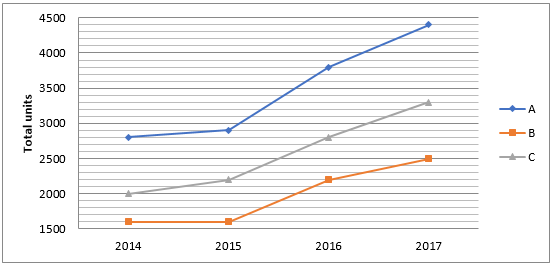Quantitative Quiz For IDBI AM/Executive 2022 13th June CHECK IN HINDI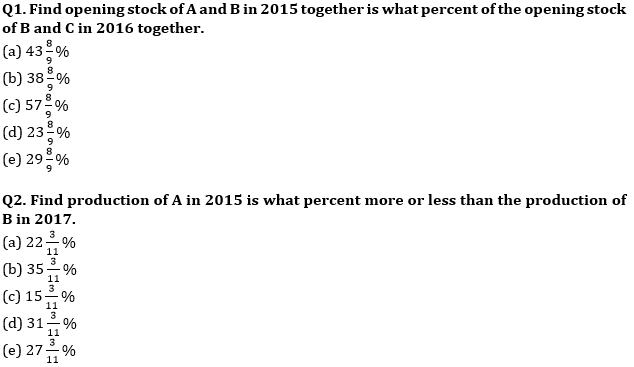Q3. Find the manufacturing cost of the company in 2018.
(a) Rs. 1,12,000
(b) Rs. 1,05,400
(c) Rs. 1,23,750
(d) Rs. 1,17,500
(e) Rs. 1,29,800

Q4. Find the ratio of the maximum possible production of A, B and C in 2014.
(a) 13 : 21 : 15
(b) 6 : 7 : 5
(c) 7 : 4 : 5
(d) 3 : 4 : 5
(e) None of the above.

Q5. Find the average of closing stock of A, B and C in 2017 is how much more than the average closing stock of A, B and C in 2015.
(a) 123
(b) 121
(c) 132
(d) 125
(e) 129

Directions (6 -10): Given below line graph shows total sold cycle of five different companies (in %) out of total manufactured cycle in two years. Total manufactured cycle by a company is same for both the years.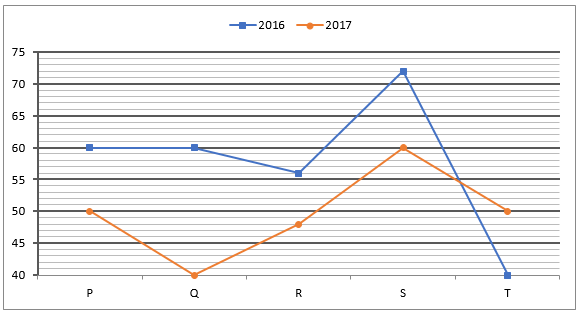Note – Manufactured cycles = Sold cycles + Unsold cycles

Q6. Find the difference between total manufactured cycles by S & T?
I. Difference between cycles sold by S in 2016 and unsold cycles by T in 2017 is 1280.
II. Difference between total unsold cycles by S in 2017 and total sold cycles by T in 2016 is 3200.
(a) Statement (I) alone is sufficient to answer the question but statement (II) alone is not sufficient to answer the questions.
(b) Statement (II) alone is sufficient to answer the question but statement (I) alone is not sufficient to answer the question.
(c) Both the statements taken together are necessary to answer the questions, but neither of the statements alone is sufficient to answer the question.
(d) Either statement (I) or statement (II) is sufficient to answer the question.
(e) Statements (I) and (II) together are not sufficient to answer the question.

Q7. If average unsold cycles in R in both the years is 7680 and total manufactured cycle by P is 40% more than total that of R, then find difference between total Sold cycles by P in both the years?
(a) 2280
(b) 2260
(c) 2200
(d) 2220
(e) 2240

Q8. If out of total unsold cycles some cycles were added as stock for next year, then find the stocks added by Q for 2017.
I. Stocks added by Q for 2017 is 62.5% less than total sold cycles by Q in 2017, while difference between total sold cycles by Q in both the years is 6400.
II. Q added 37.5% of its total unsold cycles in 2016 for 2017, while difference between total sold cycles by Q in both the years is 6400.
(a) Statement (I) alone is sufficient to answer the question but statement (II) alone is not sufficient to answer the questions.
(b) Statement (II) alone is sufficient to answer the question but statement (I) alone is not sufficient to answer the question.
(c) Both the statements taken together are necessary to answer the questions, but neither of the statements alone is sufficient to answer the question.
(d) Either statement (I) or statement (II) is sufficient to answer the question.
(e) Statements (I) and (II) together are not sufficient to answer the question.

Q9. Ratio of total manufactured cycles by R in 2016 & T in 2017 is 3 : 4 and difference between total sold cycles by R in 2016 & T in 2017 is 192. If total unsold cycles which manufactured by S in 2017 is equal to total unsold cycles by R & T in 2016 together, then find total number of unsold cycles by P in 2017, given total manufactured cycles by S in 2017 is 75% of total manufactured cycles P in same years.
(a) 3700
(b) 3680
(c) 3580
(d) 3720
(e) 3820

Q10. If total cycles manufactured by S in 2016 is 20% more than that of T in 2017 and sum of total sold cycles by both companies in the year 2016 is 2528, then find total unsold cycles by both the companies in the year 2017.
(a) 1800
(b) 1960
(c) 1600
(d) 1400
(e) 1500

Directions (11-15): Pie-chart given below shows number of person who purchased five different items during a festival season. Study the chart carefully and answer the following question.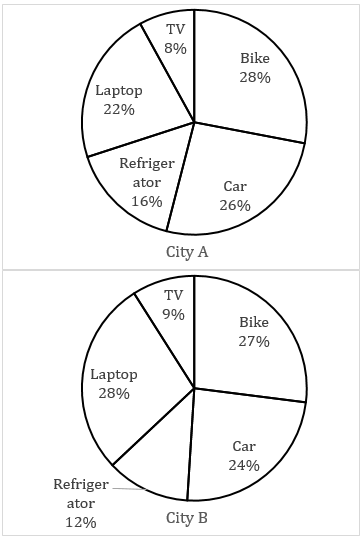Note:- Ratio of total population of city A to city B is 5 : 2.

Q11. Total number of person who purchased Laptop in city A is 945 more than that in city B, then find the total number of person who purchased car in city A and city B together?
(a) 3010
(b) 3115
(c) 3055
(d) 3085
(e) 3145

Q12. Number of females who purchased bike in city B is 25% more than number of males who purchased bike in city B, which is 25% of number of males who purchased bike in city A. If total number of person who purchased refrigerator in city B is 456, then find the number of females who purchased bike in city A.
(a) 570
(b) 1140
(c) 1824
(d) 836
(e) 912

Q13. If total number of person who purchased bike in city A and city B together is 582, then total number of person who purchased laptop and refrigerator together in city A is what percent more than total number of person who purchased laptop and refrigerator together from city B?
(a) 37.5%
(b) 50%
(c) 137.5%
(d) 150%
(e) 237.5%

Q14. If number of person who purchased bike in city B is 126 more than number of person who purchased TV in city A, then find the average number of person who purchased fridge in city A and B together?
(a) 468
(b) 364
(c) 414
(d) 428
(e) 442

Q15. Total number of person in city B who purchased fridge and TV together is how much more than total number of persons in city A who purchased TV. If it is given that total population of city B is 95 more than total number of person in city A who purchased laptop and fridge together.
(a) 13
(b) 15
(c) 17
(d) 19
(e) 21

Solutions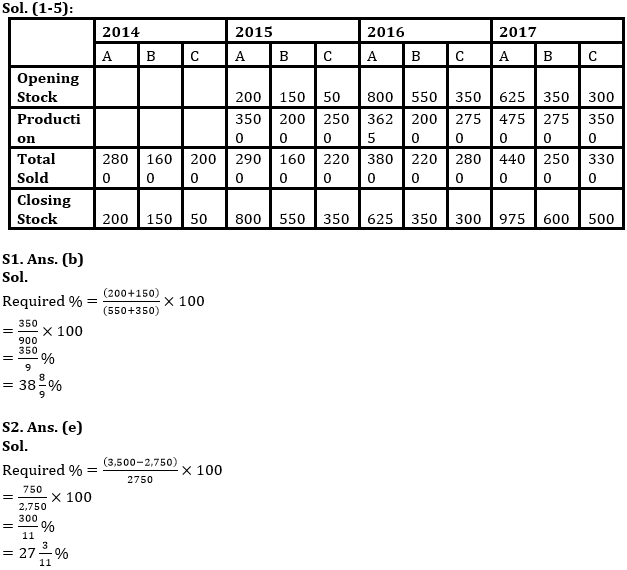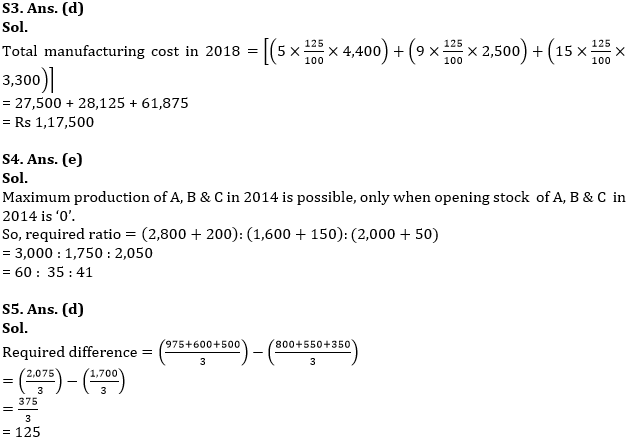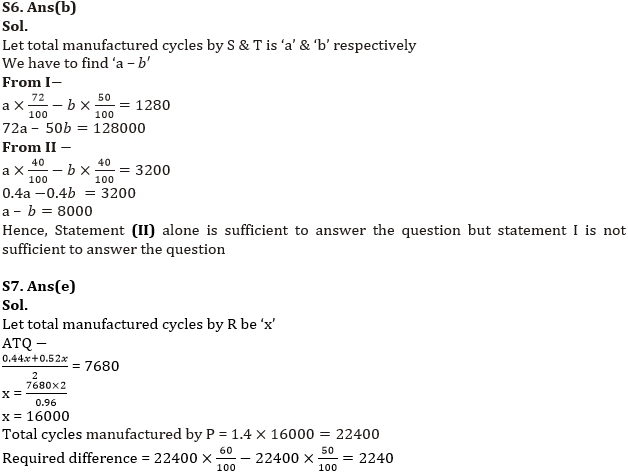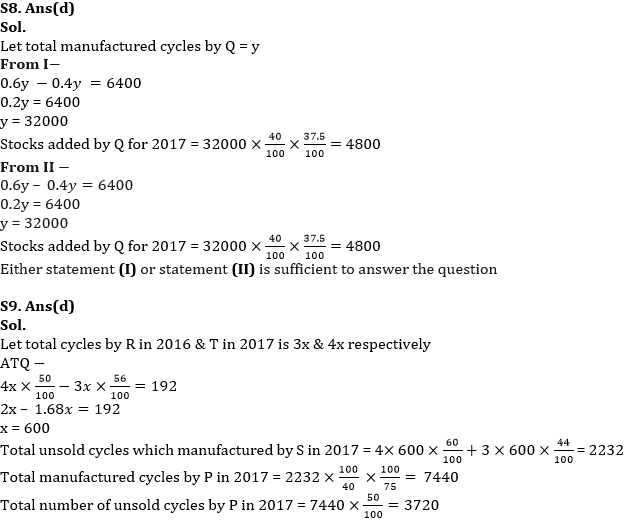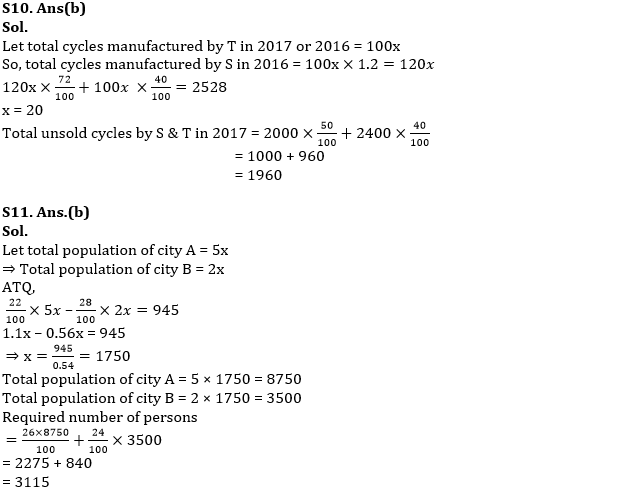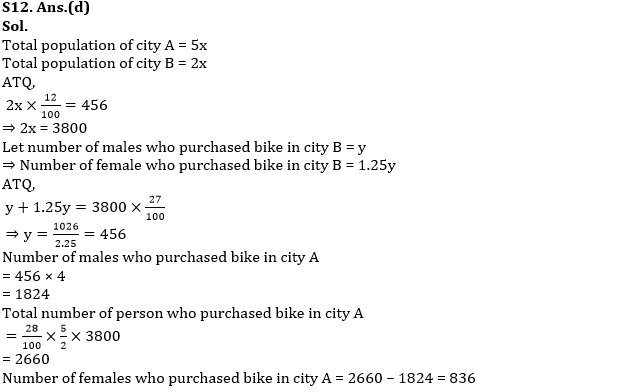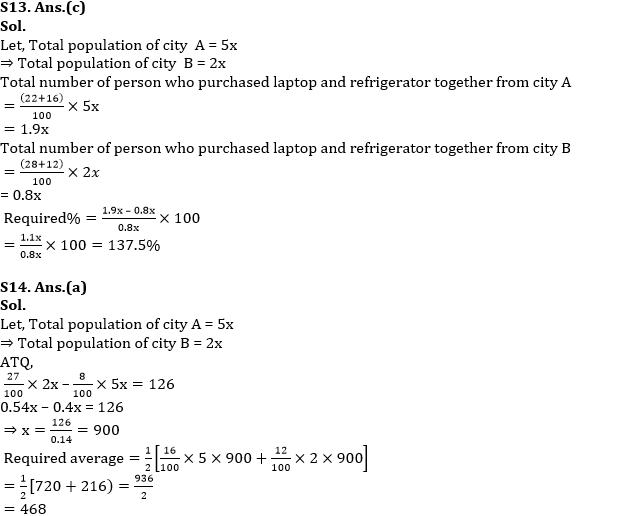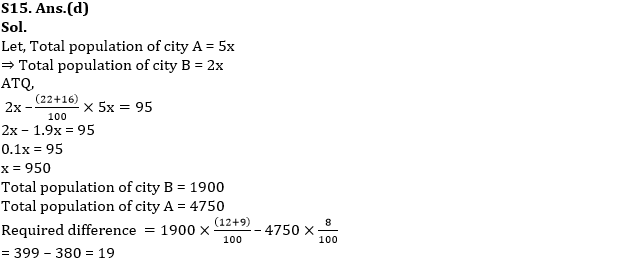#### Congratulations!